## Wednesday, June 24, 2015

### New S3 print/plot methods & new functionality for phytools ltt

I just updated the phytools function `ltt`, which computes and plots a lineage-through-time plot, even for trees that contain lineages terminating before the present day (i.e., extinct lineages).

The main changes in this version is that I created new object classes, `"ltt"` (for a single lineage-through-time plot) or `"multiLtt"` (for two or more trees supplied as an object of class `"multiPhylo"`, and then created S3 `print` and `plot` methods for these object classes.

From a practical standpoint, the main functionality added is that the arguments of `plot.default` can now be used to customize the LTT visualization. Here is a quick demo of the new methods and the new functionality of `ltt`:

``````library(phytools)
``````
``````## Loading required package: ape
``````
``````packageVersion("phytools")
``````
``````##  '0.4.58'
``````
``````## basic ltt plotting
tree<-pbtree(n=26,tip.label=LETTERS,scale=10)
obj<-ltt(tree,plot=FALSE)
## S3 print method
obj
``````
``````## Object of class "ltt" containing:
##
## (1) A phylogenetic tree with 26 tips and 25 internal nodes.
##
## (2) Vectors containing the number of lineages (ltt) and branching times (times) on the tree.
##
## (3) A value for Pybus & Harvey's "gamma" statistic of -1.1127, p-value = 0.2658.
``````
``````## S3 plot method
plot(obj,lwd=2,log.lineages=FALSE,log="y",
main="lineage through time plot")
``````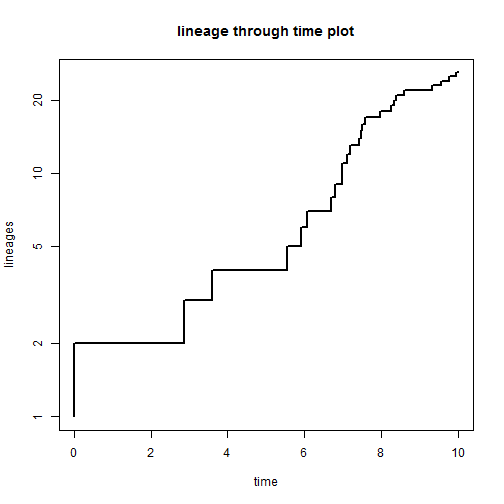``````## tree with extinction
tree<-pbtree(n=100,b=1,d=0.4,t=4)
``````
``````## simulating with both taxa-stop (n) and time-stop (t) is
## performed via rejection sampling & may be slow
##
##   2806 trees rejected before finding a tree
``````
``````obj<-ltt(tree,plot=FALSE,gamma=FALSE)
obj
``````
``````## Object of class "ltt" containing:
##
## (1) A phylogenetic tree with 143 tips and 142 internal nodes.
##
## (2) Vectors containing the number of lineages (ltt) and branching times (times) on the tree.
``````
``````plot(obj,log.lineages=FALSE,log="y",
ylab="number of lineages (log-scale)")
``````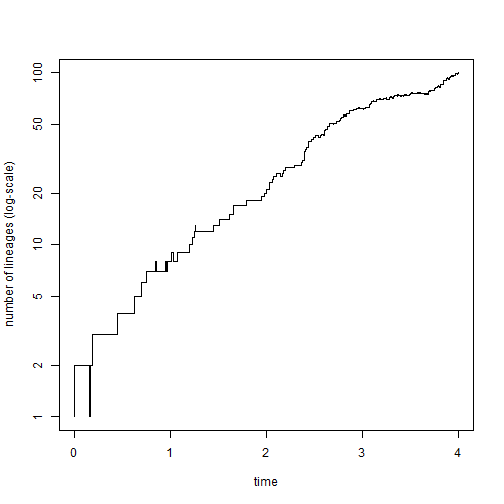``````## multiple trees
trees<-pbtree(n=40,scale=1,nsim=200)
obj<-ltt(trees)
``````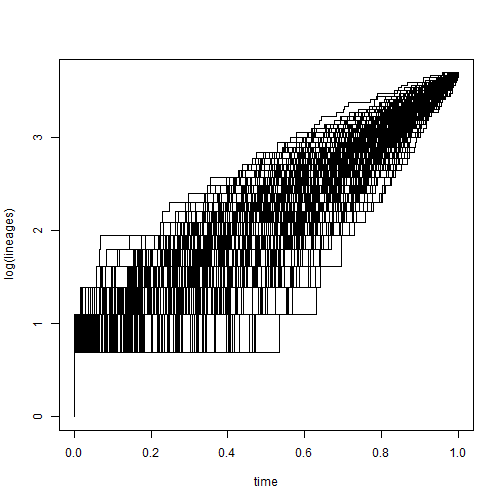``````obj
``````
``````## 200 objects of class "ltt" in a list
``````
``````## distribution of gamma
gamma<-sapply(obj,function(x) x\$gamma)
hist(gamma,main="distribution of gamma from 200 simulations")
``````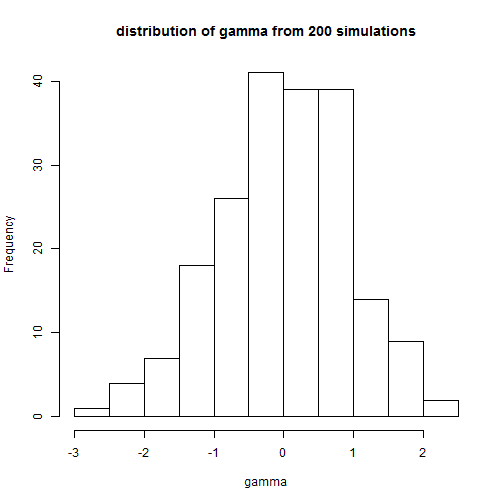``````plot(obj,log.lineages=FALSE,
main="lineage through time plots for 200 trees",
col=rgb(0,0,1,0.1)) ## plot lines with transparency
``````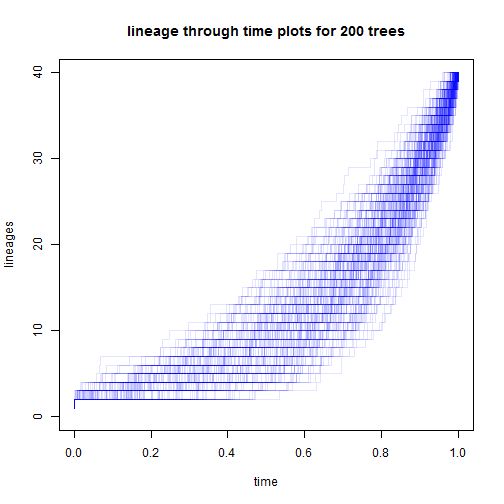The main technical challenge that I foresee is that I use the function `do.call` internally to execute `plot.default` and `lines`, depending on whether or not the lineage-through- time plot is to be added to the current graphical device. However, `plot` and `lines` take different arguments, so I can see that some users may be able to generate errors by supplying arguments that can be handled by `plot` but not by lines when the object being plotted is of class `"multiLtt"`! Please report this if you encounter it.

The code for this version of the function & associated methods is posted here, and the latest phytools package version build can be downloaded from the phytools page.

OK, that's it for now!

1.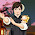Hi again,
also this one:

> plot(obj,lwd=2,log.lineages=FALSE,log="y",
+ main="lineage through time plot")
Error in xy.coords(x, y, xlabel, ylabel, log) :
'x' is a list, but does not have components 'x' and 'y'

2.Hi Liam,

I guess the first comment did send out. So I do it again. This is really awesome package! I'm running your example scripts. Everything works fine, until I'm running:
"plot(obj,log.lineages=FALSE,
main="lineage through time plots for 200 trees",
col=rgb(0,0,1,0.1)) ## plot lines with transparency
"
It gave me error:
Error in xy.coords(x, y, xlabel, ylabel, log) :
'x' is a list, but does not have components 'x' and 'y'

So it will gave me error as long as I use "plot" function. How to fix this? Thanks!

1.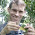Hi Miao. That error suggests to me that you do not have the most recent version of phytools installed. It should be phytools >= 0.4-60.
You can find out your current package version by using:

packageVersion("phytools")

All the best, Liam

3.Hi, Liam,

> packageVersion("phytools")
 ‘0.4.60’

Also, I have 100 of 9300 tips large tree, I used it like this:
Z100 <- ltt(trees, plot=FALSE)
plot(Z100,log.lineages=FALSE,log="y",
main="lineage through time plot",
col=rgb(0,0,1,0.1))
then it gave another error:
Warning message:
In plot.window(...) :
nonfinite axis limits [GScale(-inf,3.96848,2, .); log=1]

Sorry for the questions. I'm a brand-new beginner for R that can't handle any of error out side of script.

Thanks!

Miao

Note: due to the very large amount of spam, all comments are now automatically submitted for moderation.